# ISEE Upper Level Quantitative : How to find the length of an arc

## Example Questions

### Example Question #1 : How To Find The Length Of An Arc

The clock at the town square has a minute hand eight feet long. How far has its tip traveled since noon if it is now 12:58 PM?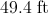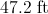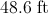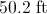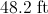Explanation:

This question is asking for the length of an arc corresponding to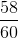of a circle with radius eight feet. The question can be answered by evaluating for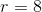: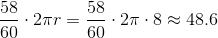### Example Question #2 : How To Find The Length Of An Arc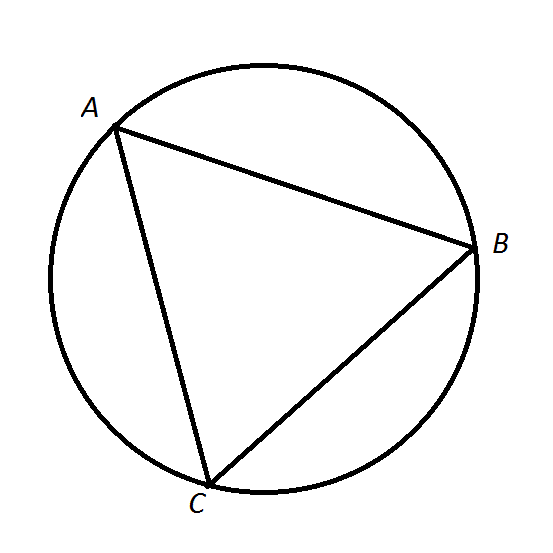Note: Figure NOT drawn to scale

Refer to the above figure.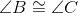Which is the greater quantity?

(a)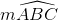(b)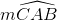It is impossible to tell from the information given

(b) is greater

(a) and (b) are equal

(a) is greater

It is impossible to tell from the information given

Explanation:

To compareand, we note that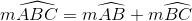and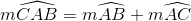We need to be able to compare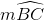and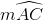. If we know which of the intercepting angles is the greater, then we know which of the arcs is greater. The intercepting angles are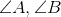, respectively. However, we are not given this relationship.

### Example Question #3 : How To Find The Length Of An Arc

A giant clock has a minute hand that is six feet long. The time is now 3:50 PM. How far has the tip of the minute hand moved, in inches, between noon and now?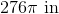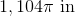The correct answer is not among these choices.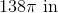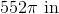Explanation:

Every hour, the tip of the minute hand travels the circumference of a circle with radius six feet, which is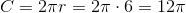feet.

Since it is now 3:50 PM, the minute hand made three complete revolutions since noon, plus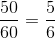of a fourth, so its tip has traveled this circumference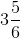times.

This is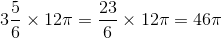feet. This is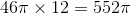inches.

### Example Question #4 : How To Find The Length Of An Arc

A giant clock has a minute hand seven feet long. Which is the greater quantity?

(A) The distance traveled by the tip of the minute hand between 1:30 PM and 2:00 PM

(B) The circumference of a circle seven feet in diameter

(A) is greater

(A) and (B) are equal

(B) is greater

It is impossible to determine which is greater from the information given

(A) and (B) are equal

Explanation:

The tip of a minute hand travels a circle whose radius is equal to the length of that minute hand, which, in this question, is seven feet long. The circumference of this circle istimes the radius, or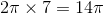feet; over the course of thrity minutes (or one-half of an hour) the tip of the minute hand covers half this distance, or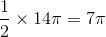feet.

The circumference of a circle seven feet in diameter istimes this diameter, or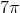feet.

The quantities are equal.

### Example Question #5 : How To Find The Length Of An Arc

A giant clock has a minute hand four and one-half feet in length. Since noon, the tip of the minute hand has traveled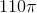feet. Which of the following is true of the time right now?

The time is between 11:30 PM and 12:00 midnight.

The time is between 12:30 AM and 1:00 AM.

The time is between 1:00 AM and 1:30 AM.

The time is between 11:00 PM and 11:30 PM.

The time is between 12:00 midnight and 12:30 AM.

The time is between 12:00 midnight and 12:30 AM.

Explanation:

Every hour, the tip of the minute hand travels the circumference of a circle, which here is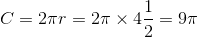feet.

The minute hand has traveledfeet since noon, so it has traveled the circumference of the circle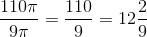times.

Since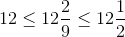, between 12 and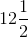hours have elapsed since noon, and the time is between 12:00 midnight and 12:30 AM.

### Example Question #6 : How To Find The Length Of An Arc

Acute triangle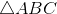is inscribed in a circle. Which is the greater quantity?

(a)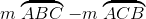(b)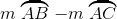(b) is the greater quantity

It is impossible to determine which is greater from the information given

(a) and (b) are equal

(a) is the greater quantity

(a) and (b) are equal

Explanation:

Examine the figure below, which showsinscribed in a circle.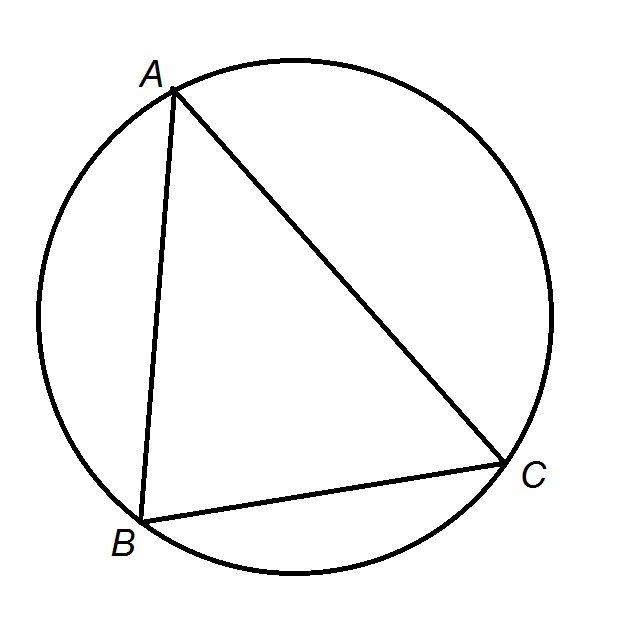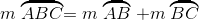and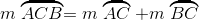Consequently,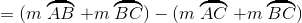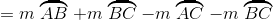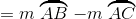The two quantities are equal.